SNAS642A June   2014  – July 2014

PRODUCTION DATA.

1. Features
2. Applications
3. Description
4. Simplified Schematic
5. Device Comparison Table
6. Pin Configuration and Functions
7. Specifications
8. Parameter Measurement Information
9. Detailed Description
1. 9.1 Overview
2. 9.2 Functional Block Diagram
3. 9.3 Feature Description
4. 9.4 Device Functional Modes
10. 10Applications and Implementation
1. 10.1 Application Information
2. 10.2 Output Clock Interface Circuit
3. 10.3 Input Detail
4. 10.4 Input Clock Interface Circuits
5. 10.5 Typical Applications
6. 10.6 Do's and Don'ts
11. 11Power Supply Recommendations
1. 11.1 Power Supply Considerations
12. 12Layout
1. 12.1 Layout Guidelines
2. 12.2 Layout Example
13. 13Device and Documentation Support
14. 14Mechanical, Packaging, and Orderable Information

• PW|16

## 10 Applications and Implementation

### 10.1 Application Information

Refer to the following sections for output clock and input clock interface circuits.

### 10.2 Output Clock Interface Circuit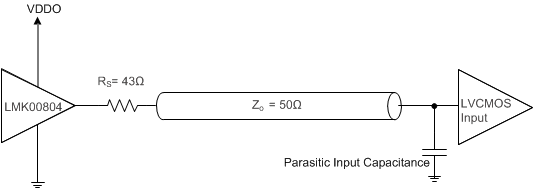Figure 9. LVCMOS Output Configuration

### 10.3 Input Detail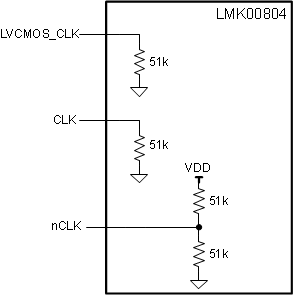Figure 10. Clock Input Components

### 10.4 Input Clock Interface Circuits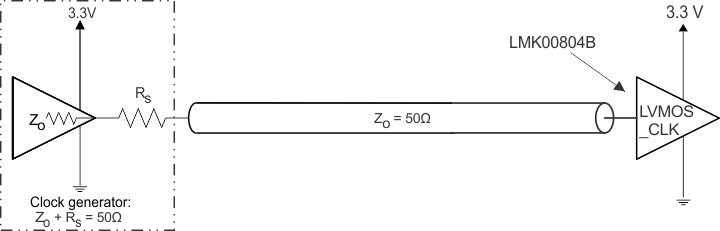Figure 11. LVCMOS_CLK Input Configuration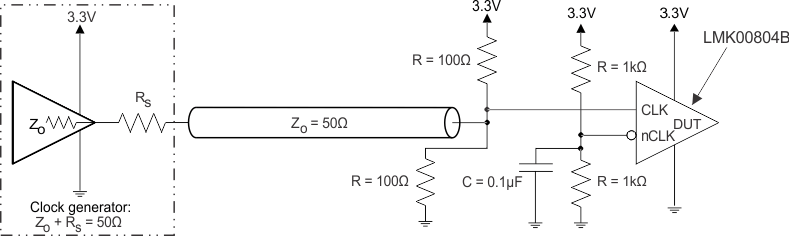1. The Thevenin/split termination values (R = 100 Ω) at the CLK input may be adjusted to provide a small differential offset voltage (50 mV, for example) between the CLK and nCLK inputs to prevent input chatter if the LVCMOS driver is tri-stated. For example, using 105 Ω 1% to 3.3 V rail and 97.6 Ω 1% to GND will provide a –60 mV offset voltage (VnCLK-VCLK) and ensure a logic low state if the LVCMOS driver is tri-stated.
Figure 12. Single-Ended/LVCMOS Input DC Configuration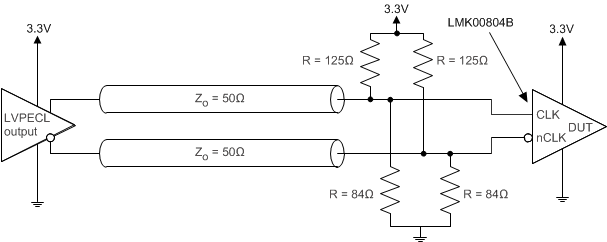Figure 13. LVPECL Input Configuration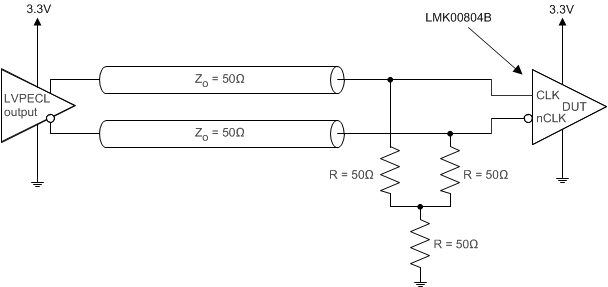Figure 14. Alternative LVPECL Input Configuration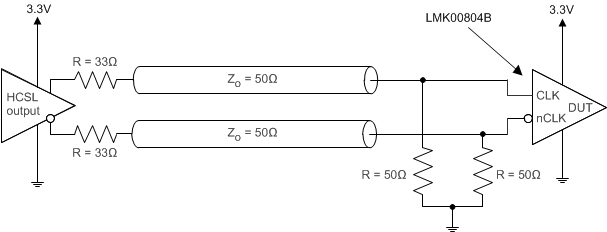Figure 15. HCSL Input ConfigurationFigure 16. LVDS Input Configuration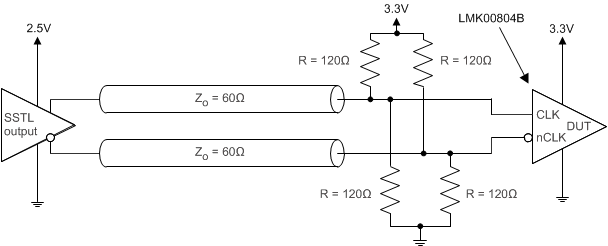Figure 17. SSTL Input Configuration

### 10.5 Typical Applications

#### 10.5.1 Design Requirements

For high-performance devices, limitations of the equipment influence phase-noise measurements. The noise floor of the equipment is often higher than the noise floor of the device. The real noise floor of the device is probably lower. It is important to understand that system-level phase noise measured at the DUT output is influenced by the input source and the measurement equipment.

For Figure 18 and Figure 19 system-level phase noise plots, a Rohde & Schwarz SMA100A low-noise signal generator was cascaded with an Agilent 70429A K95 single-ended to differential converter block with ultra-low phase noise and fast edge slew rate (>3 V/ns) to provide a very low-noise clock input source to the LMK00804B. An Agilent E5052 source signal analyzer with ultra-low measurement noise floor was used to measure the phase noise of the input source (SMA100A + 70429A K95) and system output (input source + LMK00804B). The input source phase noise is shown by the light yellow trace, and the system output phase noise is shown by the dark yellow trace.

#### 10.5.2 Detailed Design Procedure

The additive phase noise or noise floor of the buffer (PNFLOOR) can be computed as follows:

Equation 1. PNFLOOR (dBc/Hz) = 10 x log10[10^(PNSYSTEM/10) – 10^(PNSOURCE/10)]

where

• PNSYSTEM is the phase noise of the system output (source+buffer)
• PNSOURCE is the phase noise of the input source

The additive jitter of the buffer (JADD) can be computed as follows:

Equation 2. JADD = SQRT(JSYSTEM2– JSOURCE2)

where

• JSYSTEM is the RMS jitter of the system output (source+buffer), integrated from 10 kHz to 20 MHz
• JSOURCE is the RMS jitter of the input source, integrated from 10 kHz to 20 MHz

#### 10.5.3.1 System-Level Phase Noise and Additive Jitter Measurement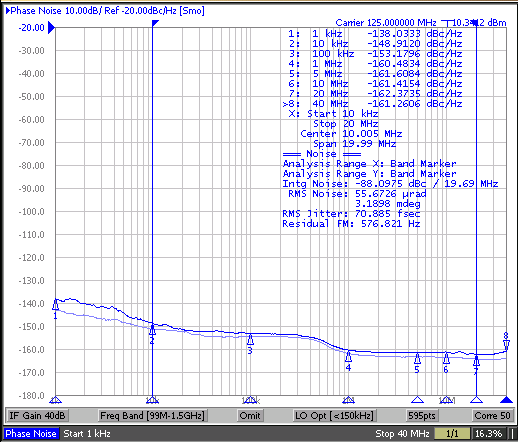Figure 18.
125 MHz Input Phase Noise (57 fs rms, Light Blue),
and Output Phase Noise (71 fs rms, Dark Blue),
Additive Jitter = 42 fs rms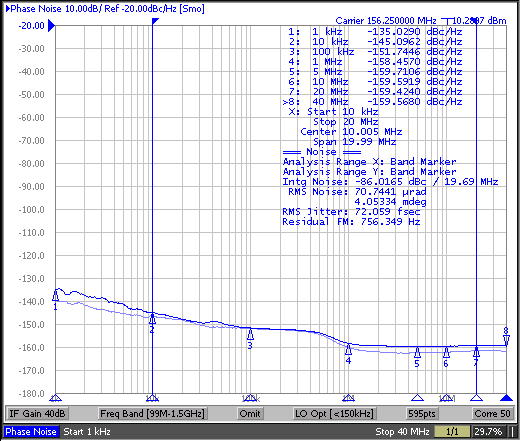Figure 19.
156.25 MHz Input Phase Noise (57 fs rms, Light Blue),
and Output Phase Noise (72 fs rms, Dark Blue),
Additive Jitter = 44 fs rms

### 10.6 Do's and Don'ts

#### 10.6.1 Power Considerations

The following power consideration refers to the device-consumed power consumption only. The device power consumption is the sum of static power and dynamic power. The dynamic power usage consists of two components:

• Power used by the device as it switches states
• Power required to charge any output load

The output load can be capacitive-only or capacitive and resistive. Use the following formula to calculate the power consumption of the device:

Equation 3. PDev = Pstat + Pdyn + PCload
Equation 4. Pstat = (IDD × VDD) + (IDDO × VDDO)

where

• IDDO,dyn = CPD × VDDO × f × n [mA]

Example for power consumption of the LMK00804B: 4 outputs are switching, f = 100 MHz,

VDD = VDDO = 3.465 V and assuming Cload = 5 pF per output:

Equation 6. PDev = 90 mW + 34 mW = 124 mW
Equation 7. Pstat = (21 mA × 3.465 V) + (5 mA × 3.465 V)= 90 mW
Equation 8. Pdyn + PCload = (2.8 mA + 6.9 mA) x 3.465 V = 34 mW
Equation 9. IDD,dyn = 2 pF × 3.465 V × 100 MHz × 4 = 2.8 mA
Equation 10. IDD,Cload = 5 pF × 3.465 V x 100 MHz × 4 = 6.9 mA

NOTE

For dimensioning the power supply, consider the total power consumption. The total power consumption is the sum of device power consumption and the power consumption of the load.

#### 10.6.2 Recommendations for Unused Input and Output Pins

• CLK_SEL, CLK_EN, and OE: These inputs all have internal pull-up (RPU) according to Table 2 and can be left floating if unused. Table 2 shows the default floating state of these inputs:
• ### Table 2. Input Floating Default States

INPUT FLOATING STATE SELECTION
CLK_SEL CLK/nCLK selected
CLK_EN Synchronous outputs enable
OE Outputs enabled
• CLK/nCLK Inputs: See Figure 10 for the internal connections. When using single ended input, take note of the internal pull-up and pull-down to make sure the unused input is properly biased. To interface a single-ended input to the CLK/nCLK input, the configuration shown in Figure 12 is recommended.
• LVCMOS_CLK Input: See Figure 10 for the internal connection. The internal pull-down (RPD) resistor ensures a low state when this input is left floating.
• Outputs: Any unused output can be left floating with no trace connected.

#### 10.6.3 Input Slew Rate Considerations

LMK00804B employs high-speed and low-latency circuit topology, allowing the device to achieve ultra-low additive jitter/phase noise and high-frequency operation. To take advantage of these benefits in the system application, it is optimal for the input signal to have a high slew rate of 3 V/ns or greater. Driving the input with a slower slew rate can degrade the additive jitter and noise floor performance. For this reason, a differential signal input is recommended over single-ended because it typically provides higher slew rate and common-mode-rejection. Refer to the “Additive Jitter vs. Input Slew Rate” plots in Typical Characteristics. Also, using an input signal with very slow input slew rate, such as less than 0.05 V/ns, has the tendency to cause output switching noise to feed-back to the input stage and cause the output to chatter. This is especially true when driving either input in single-ended fashion with a very slow slew rate, such as a sine-wave input signal.